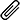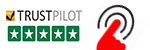High Quality, Fast Delivery, Plagiarism Free - Just in 3 Steps

## Upload Questions Details and Instructions:Select Assignment Files

## Get Assignment Help by Experts

### Plagiarism-Free, Every Time

All of our assignment solution delivers with a dedicated plagiarism report

### Excellent Subject Matter Expert

Get Supreme Assignment Help by highly Skilled Writers

### 100% Satisfaction Guaranteed

Score Higher or Your Money Back# Java program to generate a math practice test for a rst grader.

Problem 1
Math Quiz (100%) Write a Java program to generate a math practice test for a rst grader.
1. Generate 100 questions
2. Questions include multiplication, addition, subtraction, and division.
3. First grade math does not teach negative numbers. For subtraction, minuend must be greater than or
equal to subtrahend. For division problems, quotient must be an integer.
4. Operands of addition and subtraction are in the range of [1-200]. Operands of multiplication and
division are in the range of [1-99].
5. There is a Question superclass. Multiplication, Addition, Subtraction, and Division inherit Question.
6. No repeated questions.
8. Questions are randomly generated.

Sample output: Questions:
1 4 + 13 =
2 4 * 3 =
3 6 – 3 =
4 5 + 14 =
5 5 10 – 8 =
6 12 – 8 =
7 12 + 3 =
8 10 – 6 =
9 16 / 8 =
10 10 7 * 2 =
. . .
15 1 4 + 13 = 17
2 4 * 3 = 12
3 6 – 3 = 3
4 5 + 14 = 19
5 10 – 8 = 2
20 6 12 – 8 = 4
7 12 + 3 = 15
8 10 – 6 = 4
9 16 / 8 = 2
10 7 * 2 = 14
25 . . .

Homework is 100 points. 80 will re ect functionality and correctness. 20 points on your program will re ect
your programming style, documentation. If you code does not compile, you will not receive any credit.
Page 2 of 3

Anwar Mamat CMP 561 (Anwar 11:55pm): Assignment #1 Problem 1
Commenting and Documenting Code
Code must be properly commented. The main idea is that the grader should be able to understand your
code easily, not have to tear his or her hair out wondering what some statement is doing. The rst time you
have to deal with poorly commented code (if you haven’t already), you will understand how annoying it is.
In particular, the top of each code le should contain your name, the course and assignment numbers, and
a brief summary of what’s in the le. Line-by-line comments should be included as necessary to make the
code easy to read. A clear coding style, together with informative variable and function names, will reduce
the number of comments required. Obscure code or cryptic function names will cause loss of points (for bad
style) and also require more extensive comments.

What to submit
A single zip le called Assignment1 rstname lastname.zip, where rstname is your rst name, and lastname
is your last name. In this zip le, put:
1. Java source
 Instructions to compile and run of your code (include a description of command line options).
 If your solution is not perfect, explain what parts you did and what part you did not do.
 List of les submitted
 All your data and results (in plain text les).
 Anything else you want TA know
3. Submit this zip le to MoodleAsk Your Professional JAVA Programming Assignment Help?
Check out our Quick Java Assignment Help Online
Find Your Computer Science Assignment Help Online Service

We're here to instantly help! Get best assignment questions and answers help 24/7 and Earn better grades with homework